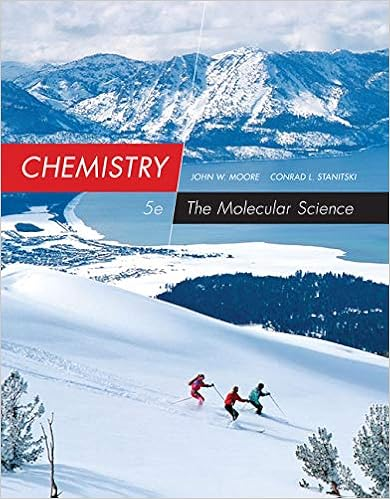# Consider the molecule below determine the molecular

• 5

This preview shows page 2 - 4 out of 5 pages.

##### We have textbook solutions for you!
The document you are viewing contains questions related to this textbook.The document you are viewing contains questions related to this textbook.
Chapter 20 / Exercise 65
Chemistry: The Molecular Science
Moore/StanitskiExpert Verified
12.Consider the molecule below. Determine the molecular geometry at each of the 2 labeled carbons.12.A)C1 =tetrahedral, C2 =linearB)C1 =trigonal planar, C2=bentC)C1 =bent, C2 =trigonal planarD)C1 =trigonal planar, C2 =tetrahedralE)C1 =trigonal pyramidal, C2 =see-saw2
##### We have textbook solutions for you!
The document you are viewing contains questions related to this textbook.The document you are viewing contains questions related to this textbook.
Chapter 20 / Exercise 65
Chemistry: The Molecular Science
Moore/StanitskiExpert Verified
13.Determine the electron geometry (eg), molecular geometry (mg), and polarity of XeF4.13.A)eg =trigonal bipyramidal, mg =trigonal bipyramidal, nonpolarB)eg =tetrahedral, mg =octahedar, polarC)eg =trigonal bipyramidal, mg =seesaw, polarD)eg =octahedral, mg =seesaw, nonpolarE)eg =octahedral, mg =square planar, nonpolar14.BCl3 is14.A)nonpolar, but contains a polar covalent bondB)polar, and contains a polar covalent bond15.How many of the following molecules are polar?XeO2SiCl2F2C2Br2SeBr615.
16.Determine the electron geometry (eg), molecular geometry (mg), and polarity of XeO3.16.
17.A molecule containing a central atom with sp3hybridization has a(n) ________ electron geometry.
18.What's the molecular shape of a molecule whose central atom has a hybrid stsae of sp318.A)tetrahedralB)square planar
19.Give the electron geometry (eg), molecular geometry (mg), and hybridization for CBr3-.19.
3
3
3
2
3
•••